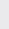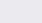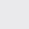Course Content

0/4

0/3

0/1

0/2

0/1

0/4

0/3

0/5

0/3

0/5

0/5

0/1

0/6

0/2

0/4

0/5

12.5 Point of Intersection of Two Lines 两条直线的交点

(1)
Find the equation of the line with gradient –4 and passes through the intersection point of lines x +2y = 3 and 2x – 5y = 15.

4x + y – 19 = 0
(2)
An isosceles triangle ABC where AB = AC. Coordinates of point A and point B are (1, –5) and (5, 2) respectively. Given that the gradient of BC isand the line perpendicular to BC and passes through A meet BC at D, find

(a) the equation of BC and equation of AD in general form,

(b) coordinate of points C and D,

(a) 2x – 3y – 4 = 0, 3x + 2y + 7 = 0

(b) (–7, -6), (–1, –2)

(c)unit

(3)
Given that 3x + 2y + 9k = 0, 2x + y – 5 = 0, x + ky + 2 = 0 are concurrent, find the value of k

–1,1,